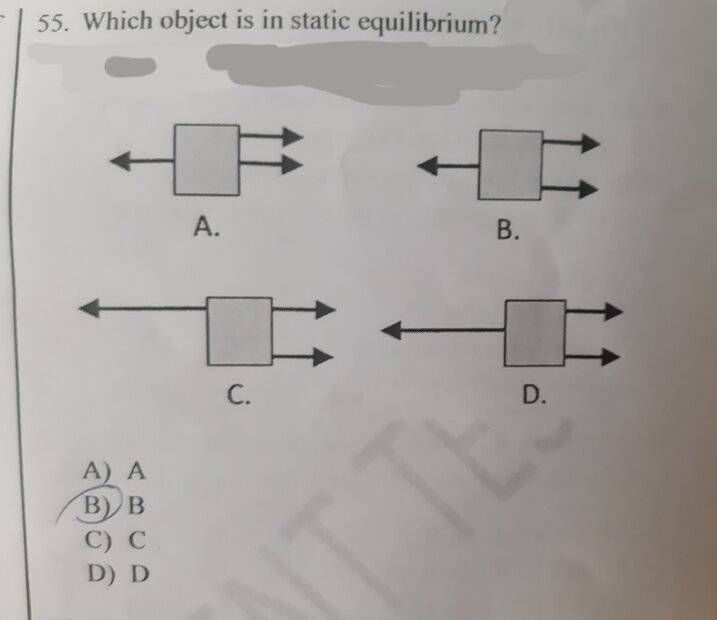# Can someone help me find which object is in static equilibrium?

• Pawn
In summary, option D has two equal forces acting on the right side of the object which balances it out.

#### Pawn

Homework Statement
The magnitudes of the forces is not given
Relevant Equations
F net = F1+F2+F3... =0Welcome!

Lnewqban said:
Welcome!
I chose B because the two forces in the right side are acting in the sides of the object while the one which is facing to the left is in the middle so i thought they will balance.
(the forces have the same magnitude in option B)

Pawn said:
I chose B because the two forces in the right side are acting in the sides of the object while the one which is facing to the left is in the middle so i thought they will balance.
(the forces have the same magnitude in option B)
Are two not more forceful than one?

PeroK said:
Are two not more forceful than one?
So the correct option is D ?

Pawn said:
So the correct option is D ?
What is your argument? Presenting that will give you better feedback than a simple yes/no.

Orodruin said:
What is your argument? Presenting that will give you better feedback than a simple yes/no.
Because the force that's acting on the left is twice that of the force that is acting in the right so the net force will be zero.

Pawn said:
Because the force that's acting on the left is twice that of the force that is acting in the right so the net force will be zero.
There's something not quite right about the way you've phrased that. What about "the single force on the left is equal in magnitude to ... forces on the right". Can you fill in the blanks?

PeroK said:
There's something not quite right about the way you've phrased that. What about "the single force on the left is equal in magnitude to ... forces on the right". Can you fill in the blanks?
The single force on the left is equal in magnitude to the sum of magnitudes of the forces on the right .

Pawn said:
The single force on the left is equal in magnitude to the sum of magnitudes of the forces on the right .
That sounds better. "Sum" is the key word here, in my opinion.

•Pawn
Two conditions have to be fulfilled in order that a rigid body is in static equilibrium:
• The sum of the external forces on the rigid object must equal zero
• The sum of the external torques on the rigid object must equal zero

•Pawn and PeroK
Pawn said:
The single force on the left is equal in magnitude to the sum of magnitudes of the forces on the right .
That leaves us both C and D. So which is it ?

•Lord Jestocost
BvU said:
That leaves us both C and D. So which is it ?
If you follow the thread back, that was in reference to option D.

It could have been better drawn, though. The two forces on the right appear to be equal in magnitude but are visibly not the same lateral displacement from the force on the left.